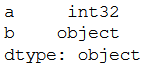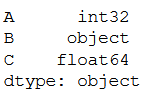Open in App
Not now

# Convert the data type of Pandas column to int

• Difficulty Level : Basic
• Last Updated : 13 Jan, 2021

In this article, we are going to see how to convert a Pandas column to int. Once a pandas.DataFrame is created using external data, systematically numeric columns are taken to as data type objects instead of int or float, creating numeric tasks not possible. We will pass any Python, Numpy, or Pandas datatype to vary all columns of a dataframe thereto type, or we will pass a dictionary having column names as keys and datatype as values to vary the type of picked columns.

Here astype() function empowers us to be express the data type you need to have. It’s extremely adaptable i.e you can attempt to go from one type to some other.

Approach:

• Import pandas
• Initialize DataFrame
• Apply function to DataFrame column
• Print data type of column

Example 1:

We first imported pandas module using the standard syntax. Then we created a dataframe with values 1, 2, 3, 4 and column indices as a and b. We named this dataframe as df. Next we converted the column type using the astype() method. The final output is converted data types of column.

Code:

## Python

 `import` `pandas as pd  `` ` `df ``=` `pd.DataFrame([[``"1"``, ``"2"``], [``"3"``, ``"4"``]],``                  ``columns ``=` `[``"a"``, ``"b"``])`` ` `df[``"a"``] ``=` `df[``"a"``].astype(``str``).astype(``int``)`` ` `print``(df.dtypes)`

Output:Example 2:

We first imported the pandas module using the standard syntax. Then we created a dataframe with values ‘A’: [1, 2, 3, 4, 5],  ‘B’: [‘a’, ‘b’, ‘c’, ‘d’, ‘e’],  ‘C’: [1.1, ‘1.0’, ‘1.3’, 2, 5] and column indices as A, B and C. We used dictionary named convert_dict to convert specific columns A and C. We named this dataframe as df. Next, we converted the column type using the astype() method. The final output is converted data types of columns.

## Python

 `import` `pandas as pd  `` ` `# sample dataframe  ``df ``=` `pd.DataFrame({``'A'``: [``1``, ``2``, ``3``, ``4``, ``5``],``                   ``'B'``: [``'a'``, ``'b'``, ``'c'``, ``'d'``, ``'e'``],``                   ``'C'``: [``1.1``, ``'1.0'``, ``'1.3'``, ``2``, ``5``] })  `` ` `# using dictionary to convert specific columns  ``convert_dict ``=` `{``'A'``: ``int``,``                ``'C'``: ``float` `}  `` ` `df ``=` `df.astype(convert_dict)  ``print``(df.dtypes)`

Output:My Personal Notes arrow_drop_up